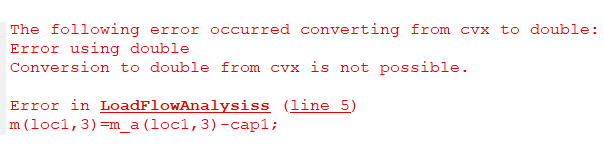# The following error occurred converting from cvx to double: Error using double Conversion to double from cvx is not possible

cvx_begin quiet
variable cap1

``````       minimize(sum(Qlosskw));
subject to
0<=cap1<=Q_max
cvx_end
``````
• and in function error for cap1:*

m(loc1,3)=m_a(loc1,3)-cap1;

The following error occurred converting from cvx to double:
Error using double
Conversion to double from cvx is not possible.

m(loc1,3)=m_a(loc1,3)-cap1;

I’m guessing that my answer Using double incorrectly. Cannot convert from cvx to double applies.

And don’t use `quiet` until you are sure everything is working well.

I used this expression but it didn’t work, actually I tried expression m(loc1,3) after variable cap1
and
expression m(loc1,3) in function but none of them worked. Could it be because of the function definition in cvx?

Show s complete reproducible code, including all the input data, and use the Preformatted text icon.applied to the entire code. Don’t have function calls unless you show us the function.

``````cvx_begin
variable cap1

minimize(sum(Qlosskw));
subject to
0<=cap1<=40;
cvx_end

m_a=m;
loc1=14;
m(loc1,3)=m_a(loc1,3)-cap1;
....
end
``````

m1 is matrix 33* 3 and line is 32* 5Where is the `expression` statement?

I tried `expression m(loc1,3)` once right after the` variable cap1` and again in the function But none of them worked

Show a complete program. with the expression statement included. If you have two versions, then show them both.

When I put` expression m(loc1,3)=m_a(loc1,3)-cap1`; inside the function
No CVX model exists in this scope.

expression m(loc1,3)=m_a(loc1,3)-cap1;

and right after the `variable cap1`

``````cvx_begin quiet
variable cap1
expression  m(14,3)
``````

The following error occurred converting from cvx to double:
Error using double
Conversion to double from cvx is not possible.

m(loc1,3)=m_a(loc1,3)-cap1;

You’re showing us bits and pieces, show us the complete program, at least up to where the CVX error message occurs. And you have not provided the part where we see what the value of `loc1` is.

And don’t use the `quiet` option.

I don’t think you can be declaring variables or expressions inside a function called from somewhere where the cvx_begiin … cvx_ebnd is. There should be another way to do what you want. Why don’t you try writing a program in which all the CVX expressions are in the one function (or script or command line).

``````global m1
global line
mm=[ 1	0         0
2	100       60
3	90        40
4	120       80
5	60        30
6	60        20
7	200       100
8	200       100
9	60        20
10	60        20
11	45        30
12	60        35
13	60        35
14	120       80
15	60        10
16	60        20
17	60        20
18	90        40
19	90        40
20	90        40
21	90        40
22	90        40
23	90        50
24	420       200
25	420       200
26	60        25
27	60        25
28	60        20
29	120       70
30	200       600
31	150       70
32	210       100
33	60        40 ];

%  line No. sending  recieving   resistance(ohm)     reactance(ohm)
%             node    node
line=[       1    1      2           0.0922              0.0470
2    2      3           0.4930              0.2511
3    3      4           0.3660              0.1864
4    4      5           0.3811              0.1941
4    5      6           0.8190              0.7070
6    6      7           0.1872              0.6188
7    7      8           0.7114              0.2351
8    8      9           1.0300              0.7400
9    9      10          1.0440              0.7400
10    10     11          0.1966              0.0650
11    11     12          0.3744              0.1238
12    12     13          1.4680              1.1550
13    13     14          0.5416              0.7129
14    14     15          0.5910              0.5260
15    15     16          0.7463              0.5450
16    16     17          1.2890              1.7210
17    17     18          0.7320              0.5740
18     2     19          0.1640              0.1565
19    19     20          1.5042              1.3554
20    20     21          0.4095              0.4784
21    21     22          0.7089              0.9373
22     3     23          0.4512              0.3083
23    23     24          0.8980              0.7091
24    24     25          0.8960              0.7011
25     6     26          0.2030              0.1034
26    26     27          0.2842              0.1447
27    27     28          1.0590              0.9337
28    28     29          0.8042              0.7006
29    29     30          0.5075              0.2585
30    30     31          0.9744              0.9630
31    31     32          0.3105              0.3619
32    32     33          0.3410              0.5302];
%      m_initrial=mm;
%      l_initial=line;

[r1,c1]=size(mm);
totalm=sum(mm(:,2));
m1=mm;
for ii=12

end
cvx_begin
variable cap1
expression  m(14,3)

minimize(sum(Qlosskw));
subject to
0<=cap1<=40;
cvx_end

m_a=m;
loc1=14;
m(loc1,3)=m_a(loc1,3)-cap1;
....
....
....

Ibrp=[abs(Ibr) angle(Ibr)*180/pi];
PL(1,1)=0;
QL(1,1)=0;

% losses
for f=1:br
Pl(f,1)=(Ibrp(f,1)^2)*R(f,1);
Ql(f,1)=X(f,1)*(Ibrp(f,1)^2);
PL(1,1)=PL(1,1)+Pl(f,1);
QL(1,1)=QL(1,1)+Ql(f,1);
end

Plosskw=(Pl)*100000;
Qlosskw=(Ql)*100000;
PL=(PL)*100000;
QL=(QL)*100000;

Voltage_Mag = vbp(:,1);
Voltage_Angle = vbp(:,2)*(pi/180);
``````

`m` in LoadFlowAnalysiss has the value `m1`from from the calling program, and `m1` is a double precision array. Then you are assigning a CvX expression into an element of it. It is a CVX expression because it includes `cap`, which is the variable `cap` from the calling program. So you did exactly what the CVX User’s Guide warned you not to do.

Try delclaring the expression you need in the calling program and pass that expression as an argument to the function. Then assign the individual element of the expression inside the function.

like that?

``````cvx_begin
variable cap1
expression  m(14,3)

minimize(sum(Qlosskw));
subject to
0<=cap1<=40;
cvx_end

Try that. You can put `whos` n the function that is called and see what it says.
Also include `m` by itself on a line with no semicolon or anything else. CVX should telll you what that is (if it is any kind of CVX thing).